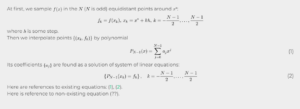# Katex/Latex with Boldgrid Page Builder

Viewing 2 posts - 1 through 2 (of 2 total)
• Author
Posts
• #39032Malick Alexandre
Guest

Im trying to write latex code inline but anytime that I start with shortcode [katex] or [latex], a weird box appear telling me to update the shortcode within the box. Even when I do the latex functions do not work.

#39049

Thank you for contacting us Malick, our team is happy to answer any questions you have about working with Latex code in the Post and Page Builder!

I located a plugin called WP QuickLaTex in the public WordPress repository that seems like it should give you the functionality you are looking for on your website.

After activating the plugin I pasted the example code from their Getting Started page into one of my page sections and the formulas rendered with the expected formatting. Here is the example code I used:

[latexpage]
At first, we sample $f(x)$ in the $N$ ($N$ is odd) equidistant points around $x^*$:
$f_k = f(x_k),\: x_k = x^*+kh,\: k=-\frac{N-1}{2},\dots,\frac{N-1}{2}$
where $h$ is some step.
Then we interpolate points $\{(x_k,f_k)\}$ by polynomial
\begin{equation} \label{eq:poly}
P_{N-1}(x)=\sum_{j=0}^{N-1}{a_jx^j}
\end{equation}
Its coefficients $\{a_j\}$ are found as a solution of system of linear equations:
\begin{equation} \label{eq:sys}